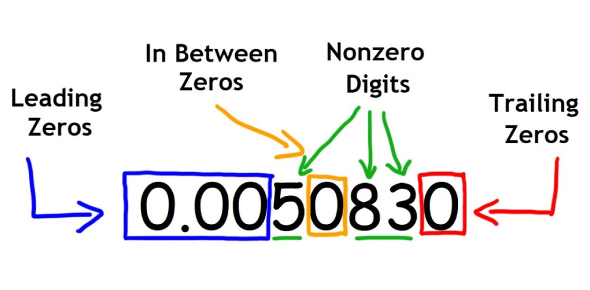# Significant Figures Quiz: Can You Score Full Marks?

15 Questions | Attempts: 53837SettingsDo you understand the concept of significant figures? Get ready for this amazing significant figures quiz. In mathematics, a significant figure refers to each of the digits of a number that is used to express it to the specified degree of accuracy, beginning from the first digit that isn't zero. For example, pi has an infinite number of significant figures but is often rounded to just three, i. E. , 3. 14. What else can you tell us about these substantial figures and what they mean? Take this sig fig quiz, and let's find out.

• 1.
How many significant figures are there in 504? Hint: All non-zero digits are significant. Zeros between digits are significant.
• A.

1

• B.

2

• C.

3

• D.

4

• 2.
How many significant figures are there in 5040 toys?  Zeros at the end of a counted quantity are not significant.
• A.

2

• B.

3

• C.

4

• D.

5

• 3.
How many significant figures are there in a substance with a mass of 504.30 grams? Hint:  Zeros at the end of a number that represents a measured quantity is a significant digit. Zeros to the right of a decimal point show how accurately a measurement is taken.
• A.

3

• B.

4

• C.

5

• D.

6

• 4.
How many significant figures are in a substance with a mass of 0.034 grams? Hint: Zeros in front of a number are not significant.
• A.

1

• B.

2

• C.

3

• D.

4

• 5.
How many significant figures are in a substance with a mass of 0.0504 grams?
• A.

5

• B.

4

• C.

3

• D.

2

• 6.
How many significant figures are in a substance with a mass of 0.07080 grams?
• A.

3

• B.

4

• C.

5

• D.

6

• 7.
How many significant figures are there in 560,890 birds?
• A.

7

• B.

6

• C.

5

• D.

4

• 8.
Add the following and answer with the correct number of significant figures: 13.4 + 2.42 = _________.
• A.

15.82 (4 significant figures)

• B.

15.8 (3 significant figures)

• C.

15.82 (2 significant figures)

• D.

15.8 (1 significant figures)

• 9.
Add the following and answer with the correct number of significant figures: 3.01 + 2.32+ 5.1 = ____________.
• A.

10.4 (3 significant figures)

• B.

10.43 (4 significant figures)

• C.

10. (2 significant figures)

• D.

10.43 (1 significant figures)

• 10.
Answer with the correct number of significant figures. 13.023x123.1 = __________.
• A.

1603.1313 (8 significant figures)

• B.

1603.1 (5 significant figures)

• C.

1603 (4 significant figures)

• D.

1603.13 (6 significant figures)

• 11.
How many significant numbers are there in 2.1390?
• A.

4

• B.

5

• C.

6

• D.

7

• 12.
How many significant numbers are there in 4.150 x 10-4?
• A.

1

• B.

2

• C.

3

• D.

4

• 13.
How many significant numbers are there in 678.02400?
• A.

5

• B.

6

• C.

7

• D.

8

• 14.
How many significant numbers are there in 0.002?
• A.

1

• B.

2

• C.

3

• D.

4

• 15.
How many significant numbers are there in 546?
• A.

1

• B.

2

• C.

3

• D.

4

## Related TopicsBack to top
×

Wait!
Here's an interesting quiz for you.